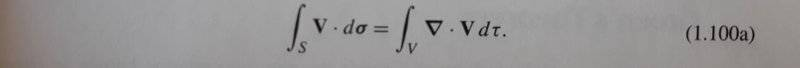# Gauss' Theorem -- Why two different notations are used?

• I
• sams

#### sams

Gold Member
In Mathematical Methods for Physicists, Sixth Edition, Page 60, Section 1.11, the Gauss' theorem is written as:In Mathematical Methods for Physicists, Fifth Edition, Page 61, Section 1.11, the Gauss' theorem is written as:Kindly I would like to know please:
1. What is the difference between the two relations?
2. What does ##\partial{V}## in Equation (1.101a) stands for? In physics, I realized that ##\partial{V}## is usually not included when Gauss' theorem is used, why is that?

Thanks a lot for your help...

#### Attachments

Last edited by a moderator:
In Mathematical Methods for Physicists, Sixth Edition, Page 60, Section 1.11, the Gauss' theorem is written as:
View attachment 231423
In Mathematical Methods for Physicists, Fifth Edition, Page 61, Section 1.11, the Gauss' theorem is written as:
View attachment 231424
Kindly I would like to know please:
1. What is the difference between the two relations?
2. What does ##\partial{V}## in Equation (1.101a) stands for? In physics, I realized that ##\partial{V}## is usually not included when Gauss' theorem is used, why is that?

Thanks a lot for your help...

Do these books not make their notation clear? The only difference is notational.

##\partial V## is sometimes used for the surface of a region ##V##. In the second equation, simply ##S## is used for the surface of the region ##V##.

•sams
The notation used by the sixth edition was to remind the reader that the left hand side is a double integral over the surface of the region and the right hand side is a triple integral over the volume of the region. They likely changed it as someone brought it to their attention or an editor schooled as a physicist took issue and decided it was best to change it.

•dextercioby and sams
•sams
Usually un physics triple or double integrals $$\int \int$$ $$\int \int \int$$
Are changed by only one simbol $$\int$$, so that is the same equation.

The simbol $$\partial v$$ means that the integral Is computed on boundary superfice of $$v$$ or on boundary of $$v$$.
$$\partial v=S$$

•sams